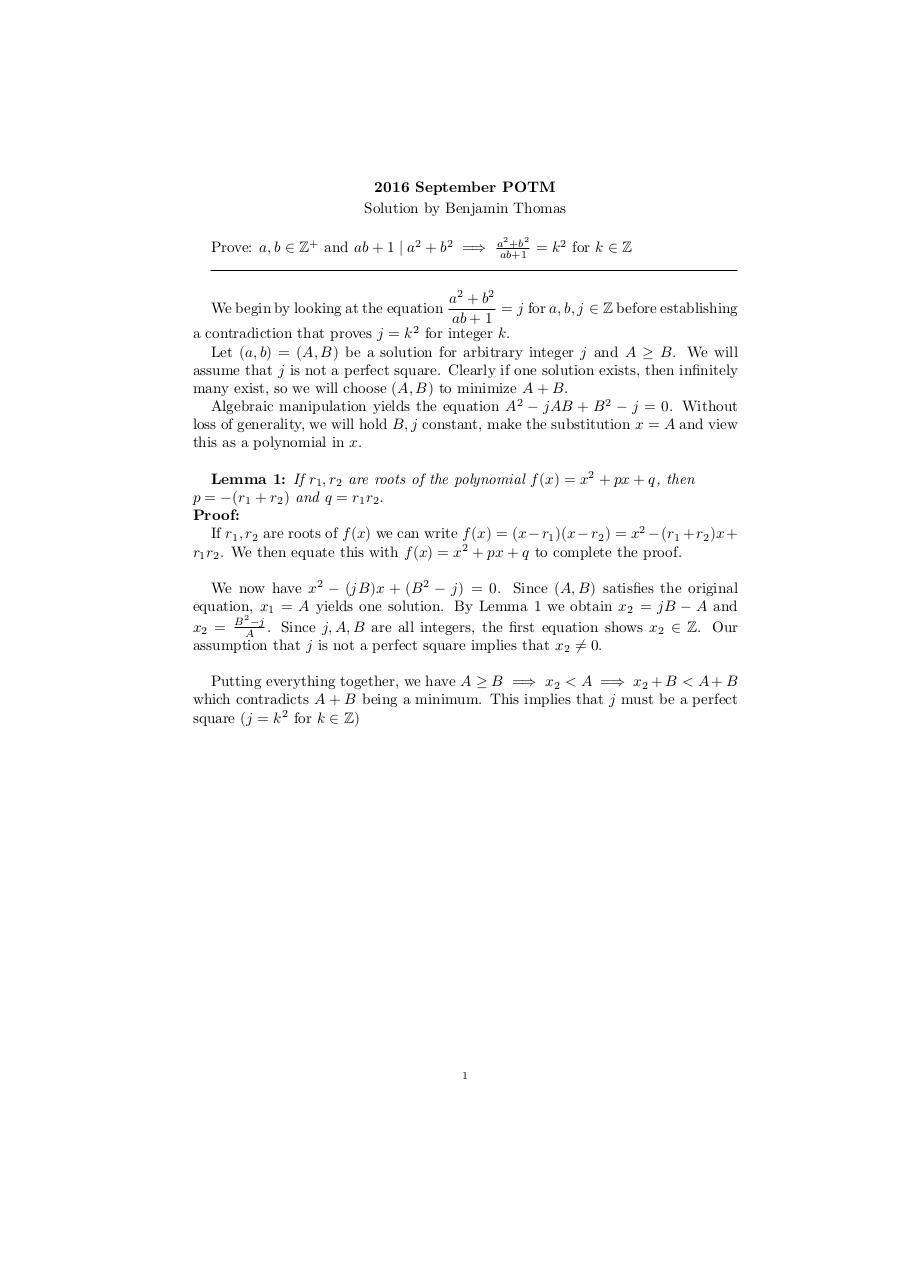# potm .pdf

### File information

Original filename: potm.pdf

This PDF 1.5 document has been generated by TeX / pdfTeX-1.40.17, and has been sent on pdf-archive.com on 24/08/2016 at 05:36, from IP address 73.185.x.x. The current document download page has been viewed 381 times.
File size: 94 KB (1 page).
Privacy: public file

potm.pdf (PDF, 94 KB)

### Document preview

2016 September POTM
Solution by Benjamin Thomas
Prove: a, b ∈ Z+ and ab + 1 | a2 + b2 =⇒

a2 +b2
ab+1

= k 2 for k ∈ Z

a2 + b2
= j for a, b, j ∈ Z before establishing
ab + 1
2
a contradiction that proves j = k for integer k.
Let (a, b) = (A, B) be a solution for arbitrary integer j and A ≥ B. We will
assume that j is not a perfect square. Clearly if one solution exists, then infinitely
many exist, so we will choose (A, B) to minimize A + B.
Algebraic manipulation yields the equation A2 − jAB + B 2 − j = 0. Without
loss of generality, we will hold B, j constant, make the substitution x = A and view
this as a polynomial in x.
We begin by looking at the equation

Lemma 1: If r1 , r2 are roots of the polynomial f (x) = x2 + px + q, then
p = −(r1 + r2 ) and q = r1 r2 .
Proof:
If r1 , r2 are roots of f (x) we can write f (x) = (x − r1 )(x − r2 ) = x2 − (r1 + r2 )x +
r1 r2 . We then equate this with f (x) = x2 + px + q to complete the proof.
We now have x2 − (jB)x + (B 2 − j) = 0. Since (A, B) satisfies the original
equation, x1 = A yields one solution. By Lemma 1 we obtain x2 = jB − A and
2
x2 = B A−j . Since j, A, B are all integers, the first equation shows x2 ∈ Z. Our
assumption that j is not a perfect square implies that x2 6= 0.
Putting everything together, we have A ≥ B =⇒ x2 &lt; A =⇒ x2 + B &lt; A + B
which contradicts A + B being a minimum. This implies that j must be a perfect
square (j = k 2 for k ∈ Z)

1#### HTML Code

Copy the following HTML code to share your document on a Website or Blog

#### QR Code### Related keywords